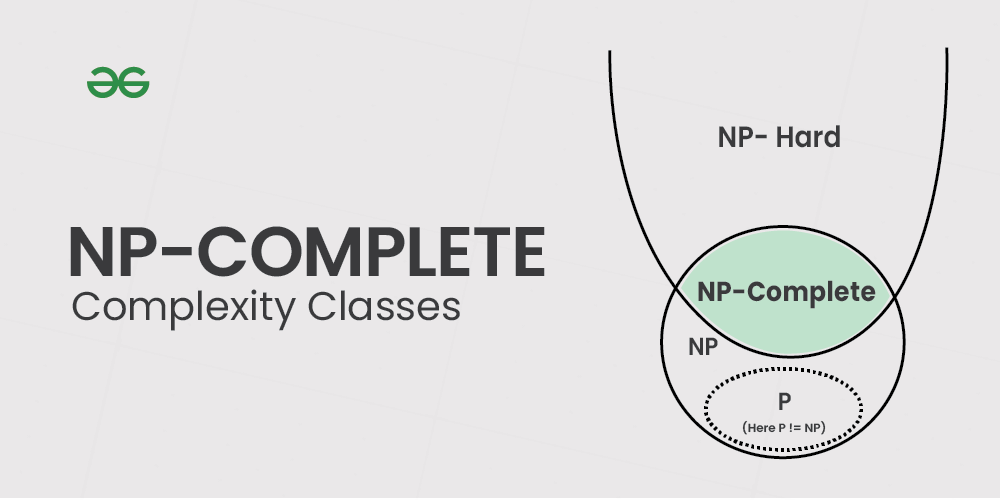Open In App

# Introduction to NP-Complete Complexity Classes

NP-complete problems are a subset of the larger class of NP (nondeterministic polynomial time) problems. NP problems are a class of computational problems that can be solved in polynomial time by a non-deterministic machine and can be verified in polynomial time by a deterministic Machine. A problem L in NP is NP-complete if all other problems in NP can be reduced to L in polynomial time. If any NP-complete problem can be solved in polynomial time, then every problem in NP can be solved in polynomial time. NP-complete problems are the hardest problems in the NP set.

A decision problem L is NP-complete if it follow the below two properties:

1. L is in NP (Any solution to NP-complete problems can be checked quickly, but no efficient solution is known).
2. Every problem in NP is reducible to L in polynomial time (Reduction is defined below).

A problem is NP-Hard if it obeys Property 2 above and need not obey Property 1. Therefore, a problem is NP-complete if it is both NP and NP-hard.NP-Complete Complexity Classes

## Decision vs Optimization Problems

NP-completeness applies to the realm of decision problems.  It was set up this way because it’s easier to compare the difficulty of decision problems than that of optimization problems.   In reality, though, being able to solve a decision problem in polynomial time will often permit us to solve the corresponding optimization problem in polynomial time (using a polynomial number of calls to the decision problem). So, discussing the difficulty of decision problems is often really equivalent to discussing the difficulty of optimization problems.
For example, consider the vertex cover problem (Given a graph, find out the minimum sized vertex set that covers all edges). It is an optimization problem. The corresponding decision problem is, given undirected graph G and k, is there a vertex cover of size k?

## What is Reduction?

Let L1 and L2 be two decision problems. Suppose algorithm A2 solves L2. That is, if y is an input for L2 then algorithm A2 will answer Yes or No depending upon whether y belongs to L2 or not.
The idea is to find a transformation from L1 to L2 so that algorithm A2 can be part of algorithm A1 to solve L1.Learning reduction, in general, is very important. For example, if we have library functions to solve certain problems and if we can reduce a new problem to one of the solved problems, we save a lot of time. Consider the example of a problem where we have to find the minimum product path in a given directed graph where the product of the path is the multiplication of weights of edges along the path. If we have code for Dijkstra’s algorithm to find the shortest path, we can take the log of all weights and use Dijkstra’s algorithm to find the minimum product path rather than writing a fresh code for this new problem.

## How to prove that a given problem is NP-complete?

From the definition of NP-complete, it appears impossible to prove that a problem L is NP-Complete.  By definition, it requires us to that show every problem in NP is polynomial time reducible to L.  Fortunately, there is an alternate way to prove it.  The idea is to take a known NP-Complete problem and reduce it to L. If a polynomial-time reduction is possible, we can prove that L is NP-Complete by transitivity of reduction (If an NP-Complete problem is reducible to L in polynomial time, then all problems are reducible to L in polynomial time).

## What was the first problem proved as NP-Complete?

There must be some first NP-Complete problem proved by the definition of NP-Complete problems.  SAT (Boolean satisfiability problem) is the first NP-Complete problem proved by Cook (See CLRS book for proof).

It is always useful to know about NP-Completeness even for engineers. Suppose you are asked to write an efficient algorithm to solve an extremely important problem for your company. After a lot of thinking, you can only come up exponential time approach which is impractical. If you don’t know about NP-Completeness, you can only say that I could not come up with an efficient algorithm. If you know about NP-Completeness and prove that the problem is NP-complete, you can proudly say that the polynomial-time solution is unlikely to exist. If there is a polynomial-time solution possible, then that solution solves a big problem of computer science many scientists have been trying for years.